IdentifiantMot de passe
Mot de passe oublié ?
7.1 What are Ufuncs?

# 7.1 What are Ufuncs?

The operations on arrays that were mentioned in the previous section (element-wise addition, multiplication, etc.) all share some features -- they all follow similar rules for broadcasting, coercion and ``element-wise operation''. Just as standard addition is available in Python through the add function in the operator module, array operations are available through callable objects as well. Thus, the following objects are available in the numarray module:

 add (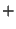) subtract (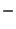) multiply (*) divide (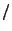) remainder (%) power (**) arccos arccosh arcsin arcsinh arctan arctanh cos cosh tan tanh log10 sin sinh sqrt absolute (abs) fabs floor ceil fmod exp log conjugate maximum minimum greater (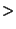) greater_equal (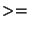) equal (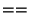) less (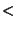) less_equal (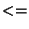) not_equal (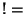) logical_or logical_xor logical_not logical_and bitwise_or (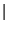) bitwise_xor (^) bitwise_not (~) bitwise_and (&) rshift (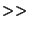) lshift (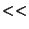)

All of these ufuncs can be used as functions. For example, to use add, which is a binary ufunc (i.e. it takes two arguments), one can do either of:

```>>> a = arange(10)
[ 0  2  4  6  8 10 12 14 16 18]
>>> print a + a
[ 0  2  4  6  8 10 12 14 16 18]
```
In other words, the `+` operator on arrays performs exactly the same thing as the add ufunc when operated on arrays. For a unary ufunc such as sin, one can do, e.g.:
```>>> a = arange(10)
>>> print sin(a)
[ 0.          0.84147096  0.90929741  0.14112    -0.7568025
-0.95892429 -0.27941549  0.65698659  0.98935825  0.41211849]
```
A unary ufunc returns an array with the same shape as its argument array, but with each element replaced by the application of the function to that element (`sin(0)=0`, `sin(1)=0.84147098`, etc.).

There are three additional features of ufuncs which make them different from standard Python functions. They can operate on any Python sequence in addition to arrays; they can take an ``output'' argument; they have methods which are themselves callable with arrays and sequences. Each of these will be described in turn.

Ufuncs can operate on any Python sequence. Ufuncs have so far been described as callable objects which take either one or two arrays as arguments (depending on whether they are unary or binary). In fact, any Python sequence which can be the input to the array constructor can be used. The return value from ufuncs is always an array. Thus:

```>>> add([1,2,3,4], (1,2,3,4))
array([2, 4, 6, 8])
```

## 7.1.1 Ufuncs can take output arguments

In many computations with large sets of numbers, arrays are often used only once. For example, a computation on a large set of numbers could involve the following step

```dataset = dataset * 1.20
```
This can also be written as the following using the Ufunc form:
```dataset = multiply(dataset, 1.20)
```
In both cases, a temporary array is created to store the results of the computation before it is finally copied into dataset. It is more efficient, both in terms of memory and computation time, to do an ``in-place'' operation. This can be done by specifying an existing array as the place to store the result of the ufunc. In this example, one can write:7.1
```multiply(dataset, 1.20, dataset)
```
This is not a step to take lightly, however. For example, the ``big and slow'' version (`dataset = dataset * 1.20`) and the ``small and fast'' version above will yield different results in at least one case:
• If the type of the target array is not that which would normally be computed, the operation will not coerce the array to the expected data type. (The result is done in the expected data type, but coerced back to the original array type.)
• Example:
```\>>> a=arange(5,type=Int32)
>>> print a[::-1]*1.7
[ 6.8  5.1  3.4  1.7  0. ]
>>> multiply(a[::-1],1.7,a)
>>> print a
[6 5 3 1 0]
>>> a *= 1.7
>>> print a
[0 1 3 5 6]
```

The output array does not need to be the same variable as the input array. In numarray, in contrast to Numeric, the output array may have any type (automatic conversion is performed on the output).

## 7.1.2 Ufuncs have special methods

 reduce( a, axis=0)
If you don't know about the reduce command in Python, review section 5.1.3 of the Python Tutorial (http://www.python.org/doc/current/tut/). Briefly, reduce is most often used with two arguments, a callable object (such as a function), and a sequence. It calls the callable object with the first two elements of the sequence, then with the result of that operation and the third element, and so on, returning at the end the successive ``reduction'' of the specified callable object over the sequence elements. Similarly, the reduce method of ufuncs is called with a sequence as an argument, and performs the reduction of that ufunc on the sequence. As an example, adding all of the elements in a rank-1 array can be done with:
```>>> a = array([1,2,3,4])
10
```
When applied to arrays which are of rank greater than one, the reduction proceeds by default along the first axis:
```>>> b = array([[1,2,3,4],[6,7,8,9]])
>>> print b
[[1 2 3 4]
[6 7 8 9]]
[ 7  9 11 13]
```
A different axis of reduction can be specified with a second integer argument:
```>>> print b
[[1 2 3 4]
[6 7 8 9]]
[10 30]
```

 accumulate( a)
The accumulate ufunc method is simular to reduce, except that it returns an array containing the intermediate results of the reduction:
```>>> a = arange(10)
>>> print a
[0 1 2 3 4 5 6 7 8 9]
[ 0  1  3  6 10 15 21 28 36 45] # 0, 0+1, 0+1+2, 0+1+2+3, ... 0+...+9
>>> print add.reduce(a) # same as add.accumulate(a)[-1] w/o side effects on a
45
```

 outer( a, b)
The third ufunc method is outer, which takes two arrays as arguments and returns the ``outer ufunc'' of the two arguments. Thus the outer method of the multiply ufunc, results in the outer product. The outer method is only supported for binary methods.
```>>> print a
[0 1 2 3 4]
>>> print b
[0 1 2 3]
[[0 1 2 3]
[1 2 3 4]
[2 3 4 5]
[3 4 5 6]
[4 5 6 7]]
>>> print multiply.outer(b,a)
[[ 0  0  0  0  0]
[ 0  1  2  3  4]
[ 0  2  4  6  8]
[ 0  3  6  9 12]]
>>> print power.outer(a,b)
[[ 1  0  0  0]
[ 1  1  1  1]
[ 1  2  4  8]
[ 1  3  9 27]
[ 1  4 16 64]]
```

 reduceat( )
The reduceat method of Numeric has not been implemented in numarray.

## 7.1.3 Ufuncs always return new arrays

Except when the output argument is used as described above, ufuncs always return new arrays which do not share any data with the input arrays.

#### Footnotes

... write:7.1
for Python-2.2.2 or later: `dataset *= 1.20' also works
Send comments to the NumArray community.